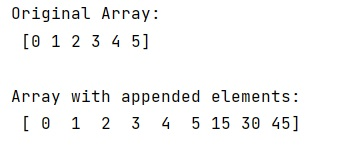# Add single element to array in numpy

Learn, how to add single element to array in numpy?
Submitted by Pranit Sharma, on December 24, 2022

NumPy is an abbreviated form of Numerical Python. It is used for different types of scientific operations in python. Numpy is a vast library in python which is used for almost every kind of scientific or mathematical operation. It is itself an array which is a collection of various methods and functions for processing the arrays.

## Adding single element to array in numpy

Suppose that we are given a NumPy array that contains some integer values and we need to add some more integer values in this array.

For this purpose, we will use numpy.append() method which creates a new array that can be the old array with the appended element.

This method does not alter the original array. However, it returns a new modified array. We should also understand the drawback of this approach, the memory is allocated for a completely new array every time it is called.

Let us understand with the help of an example,

## Python code to add single element to array in numpy

```# Import numpy
import numpy as np

# Creating a numpy array
arr = np.array([0, 1, 2, 3, 4, 5])

# Display original array
print("Original Array:\n",arr,"\n")

arr = np.append(arr,15)

arr = np.append(arr,30)

arr = np.append(arr,45)

# Display result
print("Array with appended elements:\n",arr,"\n")
```

Output: